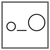Fuddlepixel coz tech is fun();

# Arrays Some cool examples

Let us discuss some cool examples to understand the concepts of arrays more easily. Creating, rotating, printing arrays, tables, average and lot more.

By Shubham Ramdeo
17 April 2016 · 4 mins read
# `c`  `newbie`That’s how we play with arrays, practically !

Welcome to the Newbie Programmer Series. In the last two parts, we have discussed the concepts behind the arrays and how to use them. In this part we will discuss some examples to learn more about arrays. So if you are new here, please check out all the previous parts (click here) so that you can easily understand what is happening below.

### Average with array formed during run time

Calculating average of some numbers is one of the easiest examples. Why I put it here in a different way is because here I have used variable array !!! WHAT ? But we have already studied in the last part that variable arrays cannot be made. Then how is it made possible ? Actually it is a trick. It is very true that once the array is created of a certain size, it cannot be change. But before creating the array, we can change the size. So in this example here, user need to set the size of the array. Then the array is created which remains fix. In the following code, program asks for total number of values. Say user enters 5. Then the program asks 5 values to be entered and then prints their average.

``````#include <stdio.h>
main()
{
int n, k, sum, avg;
printf("Enter the number of values. \n");
scanf("%d", &n);
int val[n]; //now array is created.
printf("Now enter %d values one by one",n);

for(k=0; k
``````

Study it a little by yourself. The above example is for integers, you can set it for float too. The main point to learn is how we have tricked it with array looking like variable size. If there is any trouble, please comment below.

Let’s Enjoy the output :

``````Enter the Number of values.
4
Now enter 4 values one by one.
10
20
30
40
The average of this data is 25
Press Enter to exit...
``````

### Rotating Arrays

There is no need to create any program for this. We will just look at the concept. Suppose we have a table ‘A’ and we want to rotate it to ‘B’ :

`````` (A)                      (B)
1 2 3     clockwise      7 4 1
4 5 6     rotated to     8 5 2
7 8 9                    9 6 3
``````

Here each element is moved to a different position. In a simple way, we can do it by creating a new array and then reassigning the positions. Let’s say “A[row][column]” is the original array and “B[row][column]” will become the rotated one.

`````` (A)                   (B)

0   1   2             0   1   2
-----------           -----------
0 | 1 | 2 | 3 |       0 | 7 | 4 | 1 |
-----------           -----------
1 | 4 | 5 | 6 |       1 | 8 | 5 | 2 |
-----------           -----------
2 | 7 | 8 | 9 |       2 | 9 | 9 | 3 |
-----------           -----------
``````

Comparing from the above table, you can equate like :

``````B = A;
B = A;
B = A;
B = A;
B = A;
B = A;
B = A;
B = A;
B = A;
``````

After this, the array “B” is the rotated array “A”. This rotation is also called as Transpose. Two invert a table, or rotating a table anticlockwise, instead of doing some thing again, we should make a function for a “single rotation” (as above) and then, for example, for anticlockwise rotation, rotate it clockwise three times ! Well it is a very confusing concept. But later we will learn using pointers which can make everything easy.

### Printing Multiplication Tables

While learning loops, (click here) we have already created a multiplication table program. Here let’s learn a little new thing. In that example, we have printed tables like “2 x 1 = 2… 2 x 2 = 4…”. Using loops directly without storing anything in a form of table.

Here, we will use a two-dimensional array and will print the table as it is.

The point to learn here is that how the table gets printed. So keep your eyes on the table printing code :

``````#include <stdio.h>
main()
{
int i,k,tab;
for(i=0; i<10, i++)
{
for(k=0; k<10; k++)
{
tab[i][k] = i * k;
}
}

for(i=0; i<10, i++)
{
for(k=0; k<10; k++)
{
printf("%d ",tab[i][k]);
}
printf("\n");
}

printf("Press Enter to exit...\n");
getchar();
return 0;
}
``````

Now you would have already understood how the table was formed. Let’s talk about the printing function. The concept is that the INNER loop starts printing with ROW=0, Column=0 to Column=9. When the end of a ROW is reached (column=9), the inner loops ends. And a “new line” character is printed due to the body of the second loop. The Row increases from ROW=0 to ROW=1. Same pattern is followed. All column of a row is printed then comes a new line for the second row. When all the rows are also printed, the loops ends.

I think this is enough. We have discussed some practical ways of using arrays and here we can complete our introduction of arrays. From the next part we will start discussing “structures”. My favorite as we can do programming like treating real physical objects. So please stay connected :)

##### Update me weekly ✉

Subscribe below and we’ll send you a weekly email summary of all new Code tutorials. Never miss out on learning about the next big thing.

#### Shubham RamdeoShubham Ramdeo is the founder of Fuddlepixel. He is a geek, a writer, involved with many technical and humanity projects.

Meet me!

#### Recent#### Related

`c`

`newbie`

Top